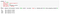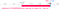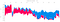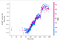# Using visualizations to unmask the machine learning black box

Posting as part of NUS CS5346 Information Visualization course.

Machine learning models can be opaque, sometimes troublingly so. Certain classes of models, such as random forests and deep neural networks, provide no clear path to understanding how a model’s inputs influences its outputs. This opacity has real-world implications. In a time where machine learning models are making consequential decisions, for example whether or not to approve a housing loan, citizens lack a way to appeal and negotiate a decision if a company is unable to explain how its model came to its conclusion. In other words, machine learning interpretability is not just an academic exercise; it is an ethical necessity.

SHAP values, proposed by Lundberg et. al, provide a solution around the black-box nature of models. SHAP values measure the extent to which input variable, such as Age or Years of Education, positively or negatively affects a models final result.

But SHAP values on their own are hard to understand. Visualisation can help. Hence, this post walks through the different visualisations of SHAP values and analyzes how they contribute to model interpretability. Along the way, we will break down the visual encodings involved in each visual and analyse how effective they are at “unboxing” a machine learning model.

# Dataset

The dataset used here is the Pima Indians Diabetes dataset, taken from Kaggle. The purpose of this dataset is to predict whether or not a group of females, all 21 years old and of Pima Indian heritage, will be diagnosed with diabetes. The input variables include features such as number of pregnancies, BMI, insulin level and age. The output variable is a binary outcome of 0 or 1. 0 indicates no diagnosis of diabetes, while 1 indicates a positive diagnosis.

We first train an XGBoost model on the data using the parameters outlined in Figure 2.Figure 2: Parameters used to train an XGBoost model on the Pima Indians Diabetes dataset. Parameters are adapted from here

Our evaluation metric for the model is the logloss, which is about 0.51794. However, at this point, we have no insight into how the model arrives at its predictions if it was to be given a new datapoint. This is where our visualisations of SHAP values become important. We will cover four visualisations here:

• Force plots
• Multi-output force plots
• Dependence plots

# Force plotFigure 3: Force plot showing how input features push model predictions away or towards the dataset’s baseline. Force plots visualise a single data point

## Purpose:

Visualise how a particular feature pushes a model’s predictions towards or away from the baseline (average of all predictions). In this case, features coloured red such as BloodPressure increase predicted values while features coloured blue such as Pregnancies decrease predicted values. The cumulative effect of all features gives the final model output, in this case a probability of 0.96 that the patient will be diagnosed with diabetes.

## Visual encodings:

Color : Red bars represent features that increase a predicted value above the baseline, while blue bars represent features that decrease a predicted value.
Length of arrow: represents the magnitude of a feature’s impact on the model’s output. In the figure above, BMI has a larger effect compared to BloodPressure.

Axis: The X-axis represents the model’s raw outputs (logits) before they are converted into probabilities that range between 0 and 1. The axis is annotated with the baseline value (average of all predictions) as well as the model’s final output.

Highlighting: Although not shown in the static figure, pop-out of the feature names and their values is shown when a user mouses over the feature in the plot.

Annotations: Each arrowed bar is annotated with the relevant feature name and value, and a legend explains what the red and blue values mean.

## Advantages of a force plot

• Users can see focus on a single data point and see how input variables influence a model’s output. This is useful when the unit of comparison needs to be one data point (eg. A single loan applicant or a single medical applicant)
• Color and size encodings make it easy to see at a glance which variables are most influential, and in what direction.
• Labels on the x-axis of baselines and predicted values convey useful information about how a model’s final output compares with the average within the dataset.

## Disadvantages of a force plot

Features can be hard to observe from the force plot if they are too numerous, or if their effects are too small. In the figure above, features in blue have a small magnitude and cannot be directly observed. A user can only observe the feature names and values when they mouse over the bar. There is no global overview of how a feature affects predictions.

# Multi-output force plot

The disadvantages of a single force plot are what leads us to our next visualisation, which is a multi-output force plot.Figure 4 Multiple force plots, one for each data point in the dataset. Force plots are flipped vertically and then ordered by similarity for purposes of comparison

## Purpose

A multi-output force plot consists of force plots for all data points. The force plots are flipped to be vertical instead of horizontal and then stacked against each other. This arrangement allows a user to have an overall picture of how features positively or negatively influence a model’s predictions. In this visualisation, the model’s outputs are the border between the red and blue areas (essentially the point at which the positive and negative forces balance each other out).

From this example, we see that, on the left, Glucose levels increase the logit values of the model (since they are in red), while towards the right, Glucose levels decrease the logit values (since they are in blue). When we mouse over the chart, we see that Glucose values on the left are high (>100) while Glucose values on the right are low (<100). This observation ties in to our knowledge of diabetes — patients with lower Glucose levels are less at risk.

## Visual encodings:

Marks: Stacking all force plots together essentially creates an area chart with red areas representing features that increase a model’s predicted value and blue areas representing features that have a negative effect on the model’s final prediction.

Channels: the x-axis encode individual data points ordered by their similarity, while the y-xis encodes the raw prediction values before they are transformed into probabilities.

Color: As with the individual force plot, red bars represent features that increase a predicted value above the baseline, while blue bars represent features that decrease a predicted value
Interactivity: Through a drop-down, users can choose between different orderings of the data points. The default is to oder data points by similarity, but users also have the option of ordering data by measures such as the output value.

## Advantages of a multi-output force plot

• Users can choose between different orderings of data points, which allows them to visualise a model’s features and outputs in different ways to understand the data better.
• Arranging all force plots together solves the problem of not being able to have a global view of how a model’s inputs affects its outputs

## Disadvantages of a multi-output force plot

It is not feasible to annotate the chart with all feature names and values. In order to see why a variable such as Glucose can have both a positive and negative effect, a user has to mouse over all Glucose values which is time consuming.

To overcome the disadvantages of a multi-output force plot, a different visualisation can be used. In the figure below, we use a dependence plot to see how the SHAP value for the Glucose feature can have both a positive and negative effect depending on its value. More specifically, lower levels of Glucose decrease the probability of being diagnosed with diabetes, while higher levels of Glucose increase the probability of diabetes.

## Purpose

See how different values of an input variable affect SHAP value of that variable.

## Visual Encodings

Marks: circles represent the intersection between a variable value (for example Glucose) and its SHAP value.

Channels : X-axis encodes the distribution of the feature in question (for example all possible values of Glucose in the dataset).
Y-axis encodes SHAP values.

Dispersion along the y-axis shows the variance in SHAP values for the same value on the x-axis. In our example, patients with the same Glucose levels may have different SHAP values associated with the Glucose feature. High dispersion is usually a clue that there are additional interactions with other features at play. To investigate this interaction further, it is possible to color the data points according to a third variable, for example Age. The use of color is explained in the following section.

Color: Color can be used to convey how the variable on the X-axis interacts with a third variable (for example Age) to cause variability in SHAP values along the y-axis. In figure 4 below, we can see that for a Glucose range of between 100 and 125, an older age tends to increase the SHAP value associated with Glucose.Figure 7: Dependence plot of Glucose levels against SHAP values, coloured by Age to convey variable interactions

## Advantages of a Dependence Plot

• A dependence plot overcomes the weaknesses associated with the multi-output force plot, namely that it is hard to see from the multi-output force plot how specific feature values are contributing to positive or negative SHAP values. With a dependence plot, a user can see at a glance how different feature values result in different SHAP values.
• Feature values usually have non-linear effects on SHAP values. A dependence plot can convey this well through the shape of the resulting scatter plot.
• Interaction effects between the variable on the x-axis and a third variable can be conveyed.
• A user can easily see the variance in SHAP values for a particular value on the X-axis. High variance on the Y-axis is usually indicative of other interaction at play that deserve further investigation.

## Disadvantages of a Dependence Plot

• Patterns in interaction effects between the X-axis variable and another feature can be sometimes be hard to interpret through the color encoding. For example in Figure 4, for Glucose values between 125 and 150, it is hard to see a discernible pattern between Age and Glucose.
• One dependence plots has to be constructed for one feature. In a model with many features, it can be different to arrange and compare all the different graphs.

# Conclusion

There is no one visualisation that can give a full view of how a machine learning model uses inputs to make predictions. Machine learning interpretability will come from creating different views of the same model and its SHAP values

## References:

I work with data in the little red dot

## More from littlereddotdata

I work with data in the little red dot